# Information in Cybernetics

The following article is from The Great Soviet Encyclopedia (1979). It might be outdated or ideologically biased.

## Information in Cybernetics

The scientific understanding of information is based on two definitions, designed for different goals (in information theory, also called the statistical theory of communication, and in the theory of statistical estimation). To these a third can also be added (currently being studied), which is connected with the concept of complexity of algorithms.

The concept of information occupies a central position in cybernetics because this science (which sets limits on our intuitive notions of information and makes them more precise) studies machines and living organisms from the viewpoint of their capability to receive certain information, store it in “memory,” transmit it through a “communication channel,” and transform it into “signals” directing their activity.

In certain cases the possibility of comparing different groups of data according to the information contained in them is as natural as the possibility of comparing plane figures according to their “area”: it may be said, independently of the method of measuring the areas, that figure A has an area which is not greater than that of B, if A can be completely placed within B (see Examples 1–3). A more profound fact—the possibility of expressing the area by a number and on this basis comparing two figures of arbitrary shape—is a result of a developed mathematical theory. Similarly, a fundamental result of information theory is the assertion that under definite and extremely broad conditions it is possible to neglect the qualitative characteristics of information and express its quantity by a number. The possibility of transmitting information through a communication channel and storing it in a memory device is determined solely by this number.

Example 1. In classical mechanics, knowledge of the instantaneous position and velocity of a particle moving in a force field gives information about its position at any future instant of time; moreover, this information is complete in the sense that the future position may be predicted exactly. However, knowledge of the particle’s energy gives information that is clearly not complete.

Example 2

The equality

(1) a = b

gives information relative to the real variables a and b. The equality

(1) a2 = b2

gives less information [since (2) follows from (1), but these equations are not equivalent]. Finally, the equality

(1) a3 = b3

which is equivalent to (1), gives the same information, that is, (1) and (3) are different forms of presenting the same information.

Example 3. The results of independent measurements of a certain physical quantity that are not error-free give information about its exact value. An increase in the number of observations increases this information.

Example 3a. The arithmetic mean of results of observations also contains certain information about the observed variable. As mathematical statistics shows, in the case of a normal distribution of probability of errors with a known variance, the arithmetic mean contains all the information.

Example 4. Let the random variable X be the result of a certain measurement. During transmission through a communication channel, X is distorted, with the result that at the receiving end the quantity Y = X + θ is received, where θ does not depend on X (in the sense of probability theory). The “output” Y gives information about the “input” X, and it is natural to expect that the larger the variance of the random error θ, the less information there is.

In each of these examples the data have been compared by the greater or lesser completeness of the information contained therein. In Examples 1–3 the meaning of this comparison is clear and leads to an analysis of the equivalence or nonequivalence of certain equations. In Examples 3a and 4 this meaning needs to be specified. This is done, respectively, by mathematical statistics and information theory (for which these examples are typical).

At the foundation of information theory lies the method, proposed in 1948 by the American scientist C. Shannon, of measuring the quantity of information contained in a random object (event, quantity, function) relative to another random object. This method leads to the expression of the quantity of information by a number. The situation can better be explained in the simplest situation, when the observed random objects are random variables that assume only a finite number of values. Let X be a random variable that assumes the values x1x2, …, xn with probabilities p1, p2, … ,pn, and let The a random variable that assumes the values yt, y2, …, ym with probabilities q1, q2, qm, qm. Then the information I(X, Y) contained in X relative to Y is defined by the formula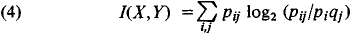where pij is the probability of the coincidence of the events X = x and Y = yj and the logarithms are taken to the base 2. The information I(X, Y)= 0 possesses a number of properties that it is natural to require of a measure of the amount of information. Thus I(X,Y)≧ 0 always, and the equality I(X,Y) = 0 is possible when and only when pij = pi, qj for all i and j, that is, when the random variables X and Y are independent. Furthermore, it is always true that I(X, Y) ≦ I(Y,Y), and equality is possible only in the case when Y is a function of X (for example, Y = X2). In addition, there exists the equality I(X,Y) = I(Y,X).

The quantity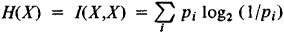is called the entropy of the random variable X. The concept of entropy is related to a number of basic concepts of information theory. The quantity of information and the entropy are connected by the relation

(5) I(X,Y) = H(X) + H(Y) - H(X, Y)

where H(X, Y) is the entropy of the pair (X,Y), that is,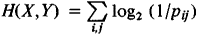The amount of entropy is indicated by the average number of binary digits necessary to distinguish between (or record) the possible values of the random variable. This circumstance allows one to understand the role of the quantity of information (4) in storage devices. If the random variables X and Y are independent, then, on the average, H(X) binary digits are required to record the value of X, H(Y) binary digits are required for the value of y, and H(X) + H(Y) binary digits for the pair (X, Y). But if the random variables X and Y are not independent, then the average number of binary digits necessary to record the pair (X,Y) turns out to be less than the sum H(X) + H(Y), since

H(X,Y) = H(X) + H(Y)— I(X, Y)

With the help of significantly more profound theorems the role of the quantity of information (4) in problems of information transmission through communication channels is explained. The fundamental information characteristic of channels, their transmission capability (or capacity), is defined by means of the concept of information.

If X and Y have a joint density distribution p(x, y), then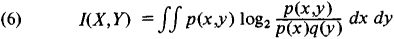where the letters p and q denote the probability densities of X and Y respectively. Moreover, the entropies H(X) and H(Y) do not exist, but there is a formula, analogous to (5),

(7) J(X,Y) = h(X) + h(Y) - h(X, Y)

where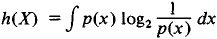is the differential entropy of X[h(Y) and H(X, Y) are defined in a similar fashion].

Example 5. Under the conditions of Example 4, let the random variables X and θ have a normal probability distribution with means equal to zero and variances equal to σ2X and σ2θ respectively. Then, as may be calculated by formulas (6) or (7):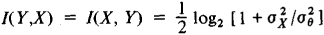Thus, the quantity of information in the “received signal” Y relative to the “transmitted signal” X tends to zero as the level of “noise” θ increases (that is, as σ1θ → ∞), and it grows without limit as the influence of the noise becomes vanishingly small (that is, as σ;2θ →0).

Special interest for communication theory is presented by the case when, under the conditions of Examples 4 and 5, the random variables X and Y are replaced by random functions (or random processes) X(t) and Y(t) that describe the change of a certain variable at the input and at the output of the transmitter. The quantity of information in Y(t) relative to X(t) for a given level of interference (“noise” in acoustical terminology) θ(t) can serve as a criterion of the quality of this same transmitter.

The concept of information is also used in problems of mathematical statistics (see Examples 3 and 3a). However, both by its formal definition and by its purpose it is distinguished from that presented above (from information theory). Statistics deals with a large number of results of observations and usually replaces their total enumeration by indicating of certain composite characteristics. Sometimes a loss of information occurs during this substitution, but under certain conditions the composite characteristics contain all the information contained in the complete set of data. (An explanation of the meaning of this statement is given at the end of Example 6.) The concept of information in statistics was introduced by the English statistician R. Fisher in 1921.

Example 6. Let X1, X2, …, Xn be the results of n independent observations of a certain variable, which are normally distributed with probability density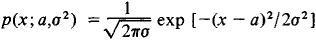where the parameters a and σ2 (the mean and the variance) are not known and must be estimated from the results of observations. Sufficient statistics (that is, functions of the results of the observations containing all the information about the unknown parameters) in this case are the arithmetic mean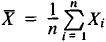and the so-called sample variance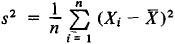If the parameter σ2 is known, then a sufficient statistic is ̄X alone (see Example 3a).

The meaning of the expression “all the information” may be explained in the following manner. Let some function of the unknown parameters ϕ = ϕ(α, σ2) exist and let

ϕ* = ϕ*(Xi, X2, …, Xn)

be some estimation of it, with the systematic errors removed. Let the quality of the estimation (its precision) be measured (as is usually done in problems of mathematical statistics) by the variance of the difference ϕ* − ϕ. Then there exists another estimation ϕ**, which does not depend on the specific values Xi but only on the composite characteristics and s2, and which is not worse (in the sense of the criterion mentioned) than ϕ*. R. Fisher also proposed a measure (average) of the quantity of information, relative to the unknown parameter, which is contained in a single observation. The meaning of this concept is revealed in the theory of statistical estimations.

### REFERENCES

Cramer, H. Matematicheskie melody statistiki. Moscow, 1948. (Translated from English.)
Van der Waerden, B. L. Matematicheskaia statistika. Moscow, 1960. (Translated from German.)
Kullback, S. Teoriia informatsii i statistika. Moscow, 1967. (Translated from English.)
IU. V. PROKHOROV